# Intro to Searching Intro to Computer Science CS

• Slides: 18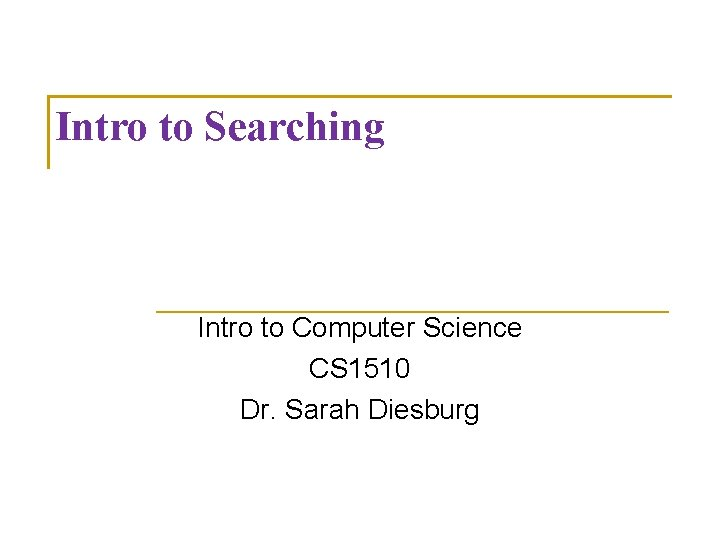Intro to Searching Intro to Computer Science CS 1510 Dr. Sarah Diesburg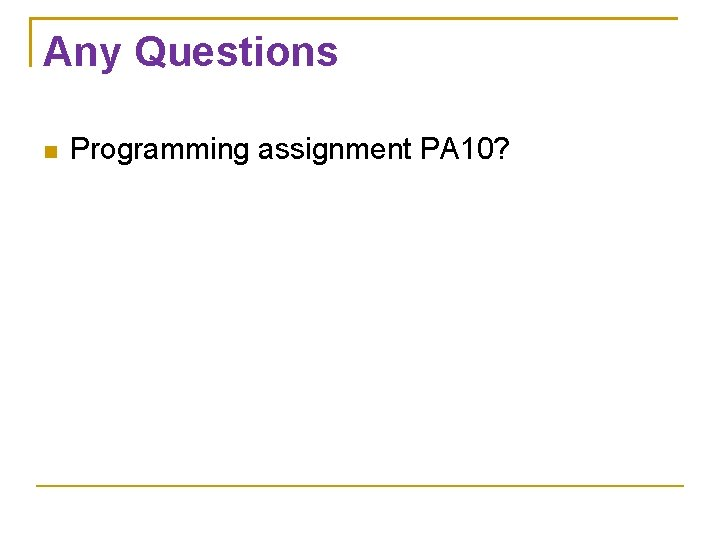Any Questions Programming assignment PA 10?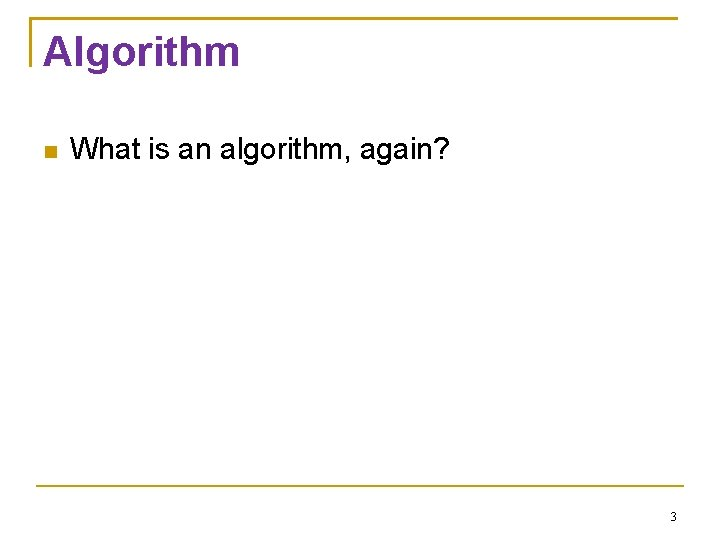Algorithm What is an algorithm, again? 3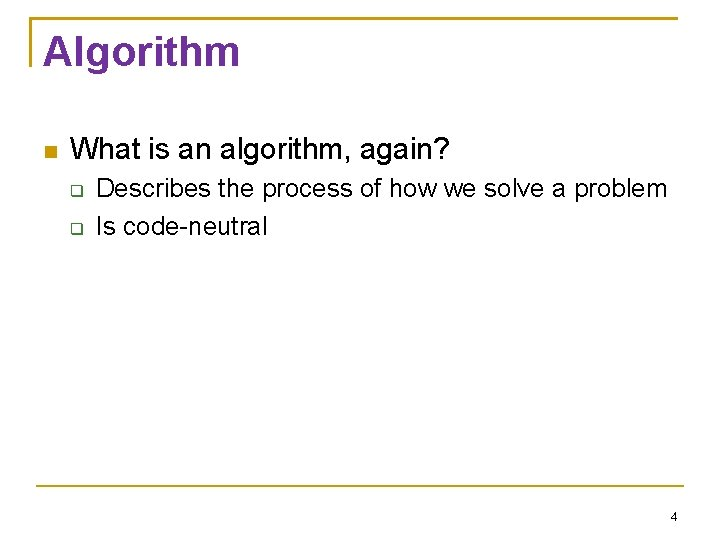Algorithm What is an algorithm, again? Describes the process of how we solve a problem Is code-neutral 4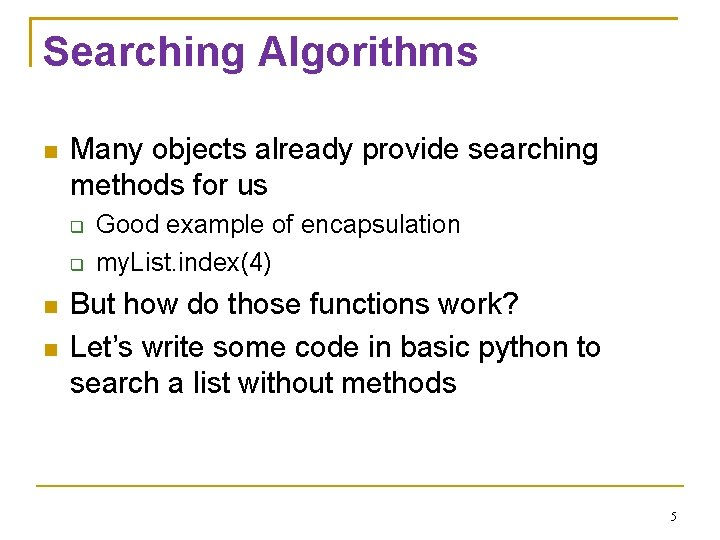Searching Algorithms Many objects already provide searching methods for us Good example of encapsulation my. List. index(4) But how do those functions work? Let’s write some code in basic python to search a list without methods 5def basic. Search(lyst, what): for item in lyst: if item==what: return True return False 6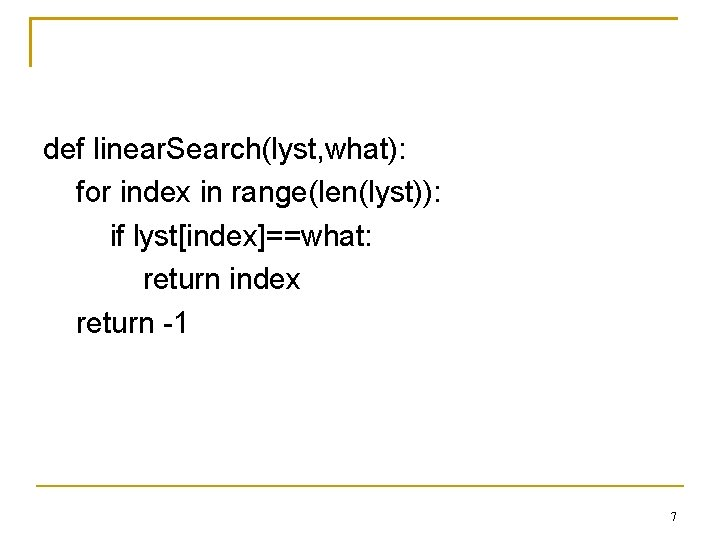def linear. Search(lyst, what): for index in range(len(lyst)): if lyst[index]==what: return index return -1 7Big “O” Notation Can we optimize our search? Big “O” notation is a analysis on how long our algorithm takes as our data set grows Typically asking for two values: On average Worst case scenario 8Big “O” Notation Based on the functions we developed Worst case scenario, how many things do we need to look at to find our number in the list? On average, how many things do we need to look at to find our number in the list? What if our list were of size 20? 9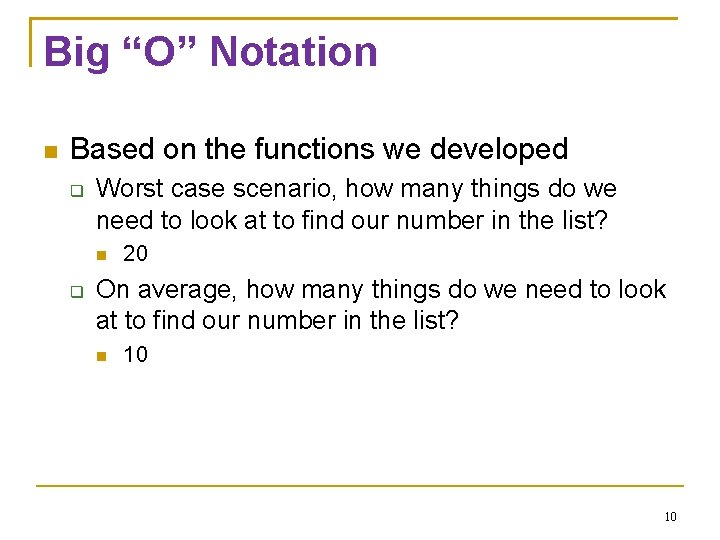Big “O” Notation Based on the functions we developed Worst case scenario, how many things do we need to look at to find our number in the list? 20 On average, how many things do we need to look at to find our number in the list? 10 10Big “O” Notation Based on the functions we developed Worst case scenario, how many things do we need to look at to find our number in the list? N, where N is the number of items in the list On average, how many things do we need to look at to find our number in the list? N/2 11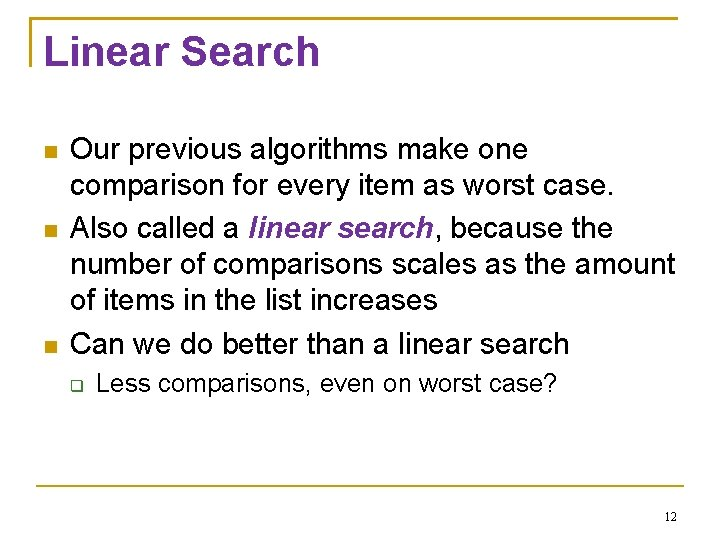Linear Search Our previous algorithms make one comparison for every item as worst case. Also called a linear search, because the number of comparisons scales as the amount of items in the list increases Can we do better than a linear search Less comparisons, even on worst case? 12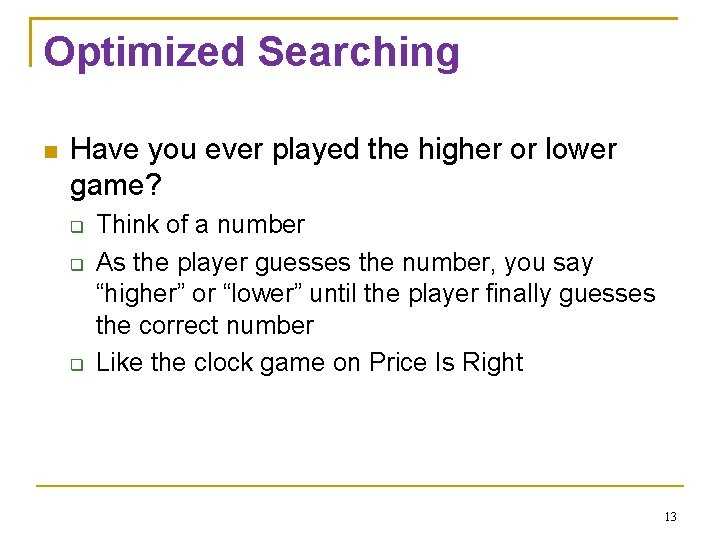Optimized Searching Have you ever played the higher or lower game? Think of a number As the player guesses the number, you say “higher” or “lower” until the player finally guesses the correct number Like the clock game on Price Is Right 13Optimized Searching One good strategy if you are the guesser is to Guess the middle number of the range If the person says “higher”, then adjust your low range bound to be your guess+1 If the person says “lower”, then adjust your high range bound to be your guess-1 Repeat 14Optimized Searching Same idea if you are looking up a vocabulary term in a dictionary You will open the book, look at the current word, and figure out if you should search lower or higher We might as well use this kind of additional information to optimize our searching 15Binary Search We can use this type of search on our list Does our list have to be sorted for this to work? Say that we have a list of 20 items What is the worst case number of comparisons? What about a list of 40 items? 16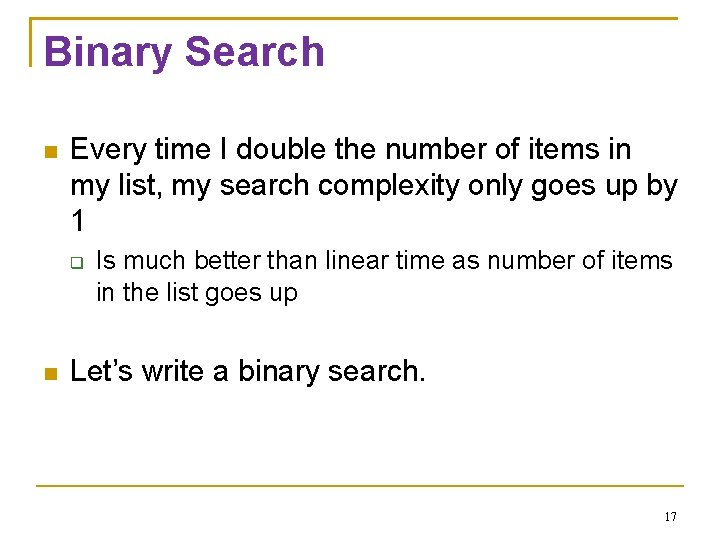Binary Search Every time I double the number of items in my list, my search complexity only goes up by 1 Is much better than linear time as number of items in the list goes up Let’s write a binary search. 17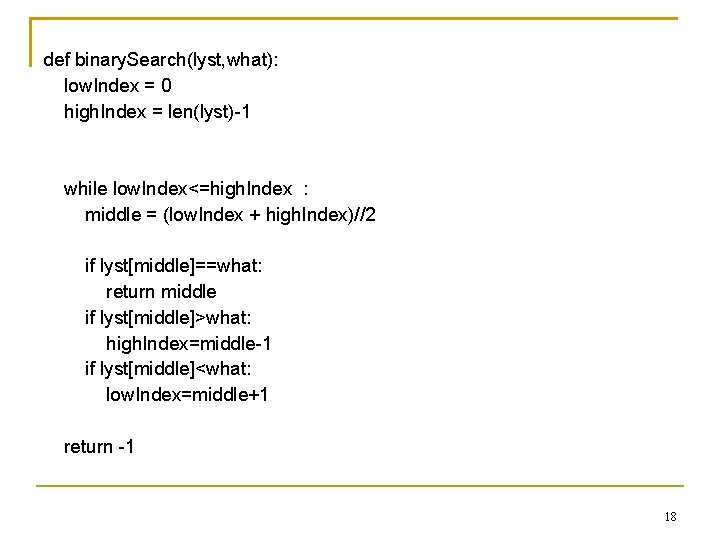def binary. Search(lyst, what): low. Index = 0 high. Index = len(lyst)-1 while low. Index<=high. Index : middle = (low. Index + high. Index)//2 if lyst[middle]==what: return middle if lyst[middle]>what: high. Index=middle-1 if lyst[middle]<what: low. Index=middle+1 return -1 18